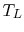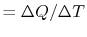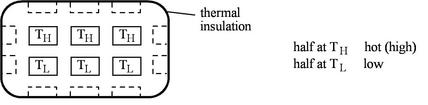Thermodynamics and Propulsion

# 4.1 Reversibility and Irreversibility in Natural Processes

We wish to characterize the direction'' of natural processes; there is a basic directionality'' in nature. We start by examining a flywheel in a fluid filled insulated enclosure as shown in Figure 4.1.A question to be asked is whether we could start with state B and then let events proceed to state A? Why or why not? The first law does not prohibit this.

The characteristics of state A are that the energy is in an organized form, the molecules in the flywheel have some circular motion, and we could extract some work by using the flywheel kinetic energy to lift a weight. In state B, in contrast, the energy is associated with disorganized motion on a molecular scale. The temperature of the fluid and flywheel are higher than in state A, so we could probably get some work out by using a Carnot cycle, but it would be much less than the work we could extract in state A. There is a qualitative difference between these states, which we need to be able to describe more precisely.

Muddy Points

Why is the ability to do work decreased in B? How do we know? (MP 4.1)

Another example is a system composed of many bricks, half at a high temperatureand half at a low temperature, as shown in Figure 4.2. With the bricks separated thermally, we have the ability to obtain work by running a cycle between the two temperatures. Suppose we put two bricks together. Using the first law we can writewhereis the heat capacity''. (For solids the heat capacities (specific heats) at constant pressure and constant volume are essentially the same.) We have lost the ability to get work out of these two bricks.Can we restore the system to the original state without contact with the outside? The answer is no. Can we restore the system to the original state with contact with the outside? The answer is yes. We could run a refrigerator to take heat out of one brick and put it into the other, but we would have to do work.

We can think of the overall process involving the system (the two bricks in an insulated setting) and the surroundings (the rest of the universe) as:

• System is changed,
• Surroundings are unchanged.

The composite system (system and the surroundings) is changed by putting the bricks together. The process is not reversible -- there is no way to undo the change and leave no mark on the surroundings.

What is the measure of change in the surroundings?

1. Energy? This is conserved.
2. Ability to do work? This is decreased.

The measurement and characterization of this type of change - of losing the ability to do work - is the subject of the second law of thermodynamics. [VW, S & B: 6.3-6.4]

UnifiedTP